Example Questions

Example Question #6 : How To Find The Surface Area Of A Cube

What is the surface area of a cube with a volume of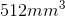? Round your answer to the nearest hundreth if necessary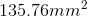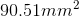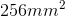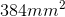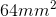Explanation:

First we need to find the side length of the cube. Do that by taking the cube root of the volume.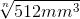=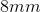Next plug the side length into the formula for the surface area of a cube: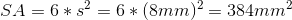Example Question #7 : How To Find The Surface Area Of A Cube

What is the surface area, in square inches, of a cube with sides measuring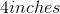?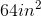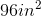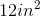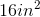Explanation:

The surface area of a cube is a measure of the total area of the surface of all of the sides of that cube.

Since a cube containssquare sides, the surface area istimes the area of a square side.

The area of one square side is sidelengthsidelength, or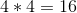in this case. Therefore, the surface area of this cube is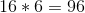square inches.

Example Question #8 : How To Find The Surface Area Of A Cube

What is the length of the side of a cube whose surface area is equal to its volume?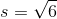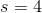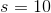There is not enough information to determine the answer.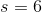Explanation:

To find the side length of a cube whose surface area is the same as its volume, set the surface area and volume equations of a cube equal to each other, the solve for the side length: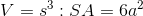Set these two formulas equal to eachother and solve for s.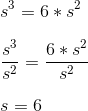Example Question #9 : How To Find The Surface Area Of A Cube

What is the surface area of a cube with a side of length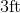?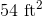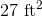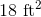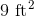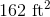Explanation:

To find the surface area of a cube with a given side length,use the formula: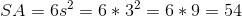Example Question #10 : How To Find The Surface Area Of A Cube

Find the surface area of a cube whose side length is.Explanation:

To find surface area of a cube, simply calculate the area of one side and multiply it by. Thus,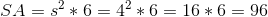Example Question #11 : How To Find The Surface Area Of A Cube

Find the surface area of a cube whose side length is.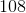Explanation:

To find surface aarea, simply multiply the area of a face by 6 since there are 6 faces. Thus,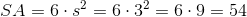Example Question #12 : How To Find The Surface Area Of A Cube

Find the surface area of a cube with side length.Explanation:

To solve, simply multiply the face area by. Thus,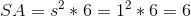Example Question #4 : How To Find The Volume Of A Cube

What is the sum of the number of vertices, edges, and faces of a cube?

18

26

None of the answers are correct

24

20

26

Explanation:

Vertices = three planes coming together at a point = 8

Edges = two planes coming together to form a line = 12

Faces = one plane as the surface of the solid = 6

Vertices + Edges + Faces = 8 + 12 + 6 = 26

Example Question #12 : How To Find The Volume Of A Cube

What is the difference in volume between a sphere with radius x and a cube with a side of 2x? Let π = 3.14

5.28x3

8.00x3

4.18x3

6.73x3

3.82x3

3.82x3

Explanation:

Vcube = s3 = (2x)3 = 8x3

Vsphere = 4/3 πr3 = 4/3•3.14•x3 = 4.18x

Example Question #13 : How To Find The Volume Of A Cube

The density of gold is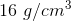and the density of glass is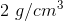.  You have a gold cube that is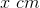in length on each side.  If you want to make a glass cube that is the same weight as the gold cube, how long must each side of the glass cube be?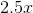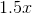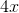Explanation:

Weight = Density * Volume

Volume of Gold Cube = side3= x3

Weight of Gold = 16 g/cm3 * x3

Weight of Glass = 3/cm3  * side3

Set the weight of the gold equal to the weight of the glass and solve for the side length:

16* x3 = 2  * side3

side3 = 16/2* x3 =  8 x3

Take the cube root of both sides:

side = 2x